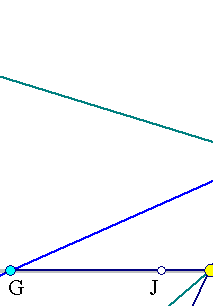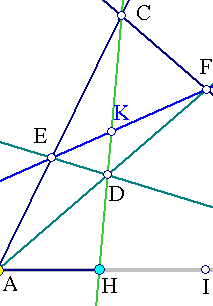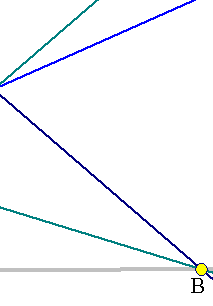##1. Harmonic conjugate points

We say that points H, G are harmonic conjugate with respect to points A, B, when all
four are on the same line and the oriented ratios:
(HA/HB) = - (GA/GB),
i. e. G, H  divide internally and externaly the segment AB into the same ratio.

We often write:
(HA/HB):(GA/GB)=-1
and say that the cross ratio (see CrossRatio0.html ):
(A,B,H,G) = -1.

The basic example of harmonic conjugate points is shown in the figure below.
First equation results  by  applying Menelaus (see Menelaus.html ) to triangle ABC and its secant (GEF).
Second equation results by  applying Ceva (see Ceva.html ) to the same triangle and point D.Menelaus for triangle ABC and secant  GEF:               (GA/GB)(FC/FB)(EA/EC)=1,
Ceva for triangle ABC and point D (see Ceva.html ):     (AH/HB)(BF/FC)(EC/EA)=-1.
Multiplying the two equations:
(GA/GB)(AH/HB)=-1, i.e. G, H are harmonic conjugate with respect to A, B.

This implies that for fixed H and D moving on line  CH  all the corresponding lines GEF pass
through the fixed point G, harmonically conjugate to H, with respect to A, B.

Notice that G, K are  also harmonically conjugate to E, F. In fact, the same arguments
apply to triangle CEF, Menelaus secant  GAB and Ceva point D.
Thus also                                               (E,F,G,K) =  -1.

There is though also another reason, why G, K are harmonically conjugate to E, F and
this is that they are cut off on line GF by a harmonic bundle of four lines
(CA, CB, CG, CH), see Harmonic_Bundle.html .

##2. Characteristic relations of the harmonic division

1) denote by {a, b, h, g} the line coordinates of the four points {A, B, H, G} on their  supporting line.
Then the relation:
HA/HB = - GA/GB   =>    HA*GB + GA*HB = 0    =>
(a-h)(b-g)+(a-g)(b-h) = 0    =>     2(ab+gh) = (a+b)(g+h).
2) Taking A as the origin of coordinates:
(a=0)   =>   2gh = b(g+h)     i.e.      2/AB = (1/AG) + (1/AH),
i.e AB is the mean harmonic of AG and AH.
3) Taking the middle  I/J of AB/GH as origin of coordinates (a+b=0, resp. g+h=0) we  get at
Newton's relations:
IA˛ = IB˛ = IG*IH,     JH˛ = JG˛ = JA*JB.
4) Relation (2) implies
2AH*AG  = AB(AH+AG) = AB*(2AJ) => AH*AG = AB*AJ.

5) Relations (3) imply:
JA/JH = JH/JB = (JH-JA)/(JB-JH) = AH/BH, and  JA/JG = JG/JB = (JA-JG)/(JG-JB)  = GA/GB.
Hence
JA/JB = (JA/JH)*(JH/JB) = (GA/GB)˛.

i.e. if G and H divide harmonically AB in ratio k, then the middle J of GH divides AB in ratio
k˛. See Apollonian_Circles.html and ApollonianBundle.html .